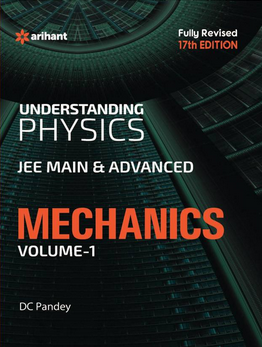# Kinematics   Share

## What is Kinematics

Kinematics is one of the most basic chapters in mechanics and you will also find a lot of use of kinematics in our day-to-day life after reading this article. For most of our lives, we have been walking, traveling from here to there and hence we are also concerned about how much time will it take for moving from one location to another. Kinematics deals with all these processes and makes our day to day life easier.

In India, most of us are cricket fans, and if you have ever noticed when the bowler bowls a ball the scoreboard also shows the speed of the ball and sometimes replays also show a possible trajectory of the ball to detect for Leg Before Wicket (LBW) test. These things are possible because Kinematics helps to understand the “motion” of the ball. Motion is nothing but a change in position. In this chapter, you will understand some basic terms like distance, displacement, speed, velocity, acceleration, etc. You should read this chapter very carefully because questions from this chapter are not only asked in 10+2 exams but also various other government exams in the General Aptitude section.

Prepare Online for JEE Main/NEET

Crack JEE 2021 with JEE/NEET Online Preparation ProgramUse of DRS to test for LBW

## Kinematics Topics

A frame of reference, Motion in a straight line- speed and velocity, Uniform and non-uniform motion, average speed and instantaneous velocity, uniformly accelerated motion, position-time, velocity-time graphs, relations for uniformly accelerated motion. Scalars and Vectors, Zero Vector, Scalar, and Vector products, Unit Vector, velocity, Resolution of a Vector, Vector Addition and Subtraction. Motion in a plane, Relative Velocity, Projectile Motion, Uniform Circular Motion.

## Overview of Kinematics

In kinematics we are not concerned about the cause of the motion, we are just concerned about the motion of the object, in simple words we don’t care how much momentum is given to the ball by the bowler, we are only interested in the motion of ball after it loses contact with the bowler’s hand. The motion of an object could be one dimensional, 2-dimensional or even 3 dimensional.

The motion of an object could also be linear or circular. In this chapter, you will get introduced to 3 equations of motion. You can solve most of the questions of kinematics using only these three equations of motion. But before going further in this chapter, I would suggest you to first develop some basic mathematical skills like differentiation, integration and also vectors. I am sure you have studied the difference between scalar and vector quantities in your high school textbooks. In this chapter, you will get introduced to a frame of reference. The frame of reference is a coordinate system. There are plenty of coordinate systems in use, but the Cartesian coordinate system, comprising of three mutually perpendicular axes, is most common. In reality, nothing is stationary, the concept of stationary is used with the frame of reference. For example, a building is stationary only if you are observing it on earth but if you see this building from the moon it is not stationary as the earth is also rotating. Sounds interesting? Now you can prank your classmates with this funda of a frame of reference.

In the beginning, you will often make mistakes sometimes also or subtraction of quantities for example if a ball is thrown vertically downwards from a building with velocity “u” then what will be the final velocity after time t? Will it be equal to “u+gt” or “u-gt”? So to minimize these type of confusions you should always use vector in vector quantities. Questions from this chapter are tricky when they are in graphical form. You should get familiar with basic equations of a line, parabola to get the solution.

Let’s assume your school bus starts at 7:00 AM then how much distance will it cover till 7:45 AM?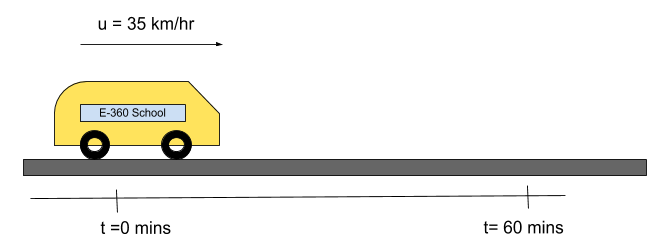Solution :

Given## Formulas for Kinematics

••••Equations of motion

1.2.3.## How to prepare Kinematics?

Before solving questions you should have a good command over basic calculus, you should be able to differentiate and integrate functions. For getting a good hold on the concepts you should first solve the question from one-dimensional motion then only solve the 2-dimensional motion. Also, learn the basics of making graphs. After reading a topic take a few mock tests on that particular topic to get the strength on the topic. Sometimes questions asked from relative motion, projectile motion and river problem are difficult hence practice more on these topics.

## Notes for Kinematics

• With the help of differentiation and integration, you can always find functions of x,v, a from one given function. Look at the below diagram to understand it. This trick is very important because it will be used in many questions.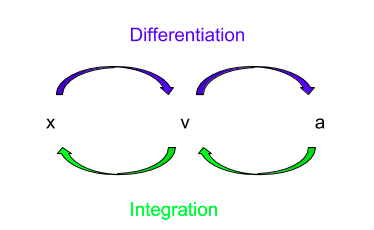• Make graphs yourself for various equations which you come across during solving questions. E.g. x-t graph, v-t graph, a-t graph, v-x graph and a-x graph etc.

• Use vector notation in vector quantities, directions are very important to avoid silly mistakes.

• Do not only memorize formulas, first prove them yourself.

• For motion in 2-D or 3-D always break (split) the motion into components along axes.

• There is a general tip for all type of questions while practicing questions always write what quantities are given in the question and what you have to find.

## Books For Kinematics

You will find easy to moderate level questions in NCERT books, please solve them first. Then you can do questions from Understanding Physics by D.C. Pandey or H.C. Verma. Both of these books have good quality questions. But apart from these books, you should also give topic-wise online mock tests. Our platform will provide you with videos for all topics, articles in simple language to make learning easy and a variety of questions in mock tests.

## Physics Chapter wise Notes For Engineering and Medical Exams

 Chapters No. Chapters Name Chapter 1 Physics and Measurement Chapter 3 Laws of motion Chapter 4 Work Energy and Power Chapter 5 Rotational Motion Chapter 6 Gravitation Chapter 7 Properties of Solids and Liquids Chapter 8 Kinetic theory of Gases Chapter 9 Thermodynamics Chapter 10 Oscillations and Waves Chapter 11 Electrostatics Chapter 12 Current Electricity Chapter 13 Magnetic Effects of Current and Magnetism Chapter 14 Electromagnetic Induction and Alternating currents Chapter 15 Electromagnetic Waves Chapter 16 Optics Chapter 17 Dual Nature of Matter and Radiation Chapter 18 Atoms And Nuclei Chapter 19 Electronic devices Chapter 20 Communication Systems Chapter 21 Experimental skills

### Topics from Kinematics

• Motion in a straight line: Position-time graph, speed, and velocity. ( JEE Main ) (18 concepts)
• The frame of reference. ( JEE Main ) (1 concepts)
• Motion in a plane ( JEE Main ) (1 concepts)
• Scalars and Vectors, Vector addition and Subtraction, Zero Vector, Scalar and Vector products, Unit Vector, Resolution of a Vector. ( JEE Main ) (22 concepts)
• Uniform and non-uniform motion, average speed and instantaneous velocity ( JEE Main ) (6 concepts)
• Relative Velocity ( JEE Main ) (10 concepts)
• Uniformly accelerated motion, velocity-time, position-time graphs, relations for uniformly accelerated motion. ( JEE Main ) (13 concepts)
• Uniform Circular Motion ( JEE Main ) (8 concepts)
• Projectile Motion ( JEE Main ) (21 concepts)
• Basics of Kinematics ( JEE Main ) (5 concepts)
• 2D motion ( JEE Main ) (9 concepts)
• Physical quantities in kinematics ( JEE Main ) (5 concepts)

### Important Books for Kinematics

•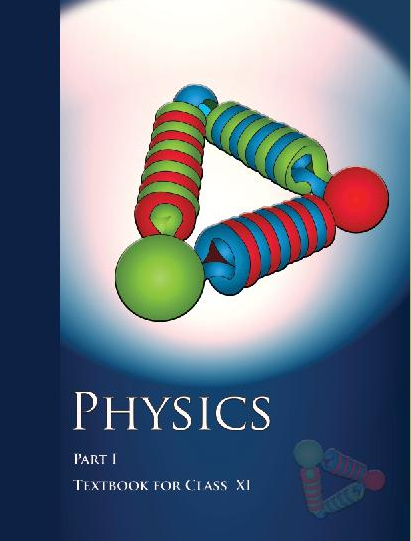•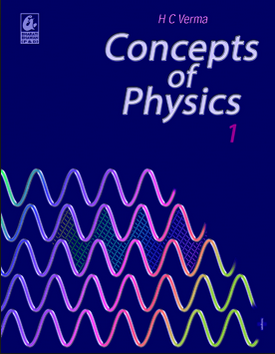•